## Wednesday, February 4, 2009

### Irodov Problem 1.266The velocity of the belt at different points is shown in the figure beside. At each point, the velocity of the belt is a sum of two motions i) its translational velocity v as the entire conveyer moves forward and ii) the motion of the belt relative to the conveyer which is v in a direction tangential to the belt at any given point on the belt. Let the mass per unit length of the belt be p.

In section CD, the belt moves from C to D with velocityrelative to the conveyer while the conveyer itself moves in the direction D to C with a velocity v. So the net velocity of this section of belt is v - v = 0. In other words the lower part of the belt is stationary! This part of the belt has no kinetic energy.

In section AB, the belt moves from A to B with a velocity v relative to the conveyer while the conveyer itself moves with v in the direction from A to B. Thus, the net velocity of this part of the belt is v + v = 2v. If the kinetic energy of this part of the belt is given by,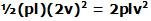In sections AD and BC, the direction of the velocity of the belt with respect to the conveyer is always v but in a direction tangential to the belt. Let the radius if the circular part be r.

In section AD, if we consider an infinitesimally small piece of the belt that subtends an angle, then its mass will be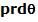. The net velocity of this point will be,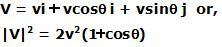The kinetic energy of this infinitesimally small part of belt is given by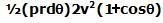.
The net kinetic energy of this part of the belt can be found as,Similarly, in section BC, for an infinitesimally small piece of the belt that subtends an angle, its velocity will be,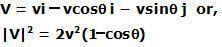Thus, the kinetic energy of this piece is given by,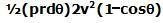The net kinetic energy of this piece of the belt is obtained as,Adding the kinetic energies of all the parts of the belt we have the total kinetic energy as,